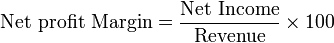# Profit Margin

It is calculated by finding the net profit as a percentage of revenue;where Net Income = Revenue – Cost
A low profit margin indicates a low margin of safety: higher risk that a decline in sales will erase profits and result in a net loss, or a negative margin.Profit margin is an indicator of a company’s pricing strategies and how well it controls costs. Differences in competitive strategy and product mix cause the profit margin to vary among different companies.

eg. Google has a net income (quarterly) of 2,785,000 and a revenue of 12,214,000, therefore this gives a net profit margin of (2,785,000/12,214,000)*100= 22.8%